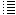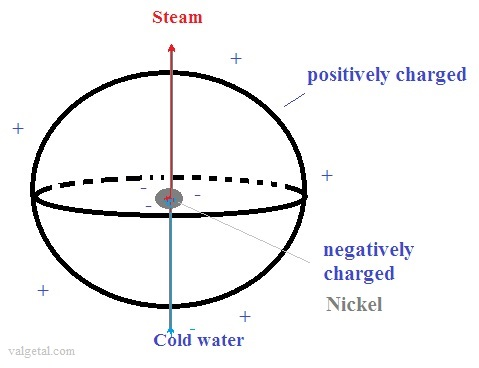1.  Breaking the Coulomb barrier with interfering microwaves?Just some idea's.... (brainstorming) 1e ideaGenerate waves (microwaves) and let them propagate in a circular waveguide. The radius of the waveguide has such a size that the waves interfere inside the waveguide and reinforce each other.

If there would be almost no losses, then the amplitude of the waves would increase and increase until almost infinite??

In the wave guide there is hydrogen gas/nuclei inside and some rigid crystal structure of, for example, nickel. Or the hydrogen is introduced later one when the waves have already a very high amplitude.

The nuclei of the hydrogen gas would be accelerated and collide with the nickel nuclei??

Could the Coulomb barrier be broken?

Possible nuclear reaction:

5828Ni + 11H  -> 5927Cu + 01e+ ( -> unstable isotope of Cu, will decay further)

57,9353 u + 1,0078 u  -> 58,9395 u + 0,00055 u   (difference left - right = 3,05. 10 -3 u)

(u = 1/12.(massa atom 126C) = 1,49.10-10 J = 1,66.10-27 kg)

So there will be liberated 3,05. 10 -3 u =  4,55.10-13 J  =  2,8 .10 eV  *  of energy (with one atom of Ni).

1 gram of Nickel => 0,001 / (57,9353. 1,66.10-27)  = 1,04.1022 atoms => 1,04.10 22 . 4,55.10-13 J = 4,73.109J = about the quantity of heat to evaporate 1780 ltr water of 4º C.

(specific heat of water is 4186 J/kgºC , and heat of vaporization of water is 2256 kJ/kg,-> 4187x96+2,26.106 ->2,66.106 J/kg )

Electrostatic potential energy:

Ucoul =     k. Z 1. Z 2 .  e2 / r

k = Coulomb's constant = 8,9876.109 Nm2 C -2
Z i = atomic numbers
e = 1,60217653.10-19 C

The strong nuclear force works until ± 2 fm = 2.10 -15 m

Z Ni  = 28
Z 1    = 1         = >   Ucoul =   3,2.10 -12  Nm =    3,2.10 -12   J  =  20.10 6  eV    ; this is much more then the energy
* liberated by the nuclear reaction .

But at the core of the Sun protons require energies of only  3–10 keV to overcome their mutual repulsion (due to quantum tunneling).

Let's suppose 10 keV is needed here: 10 keV = 10.103 .1,6.10-19 J = 16.10-16 J , this is a lot smaller then the energy  * liberated by the nuclear reaction .

Would it be possible to generate standing microwaves with enough intensity??

I don't think so...

The electrical force a H+ experiences because of the oscillating electric field of a microwave is about  2,4.10-16 N
(calculations are in my notebook)

To break the Coulomb barrier between a Ni nucleus and a H+ a force is needed of 1611 N !

Suppose the circle circumference of the wave guide is 2.π mtr and there are not losses.

Then 4400 year is needed for the waves inside the wave guide to form an amplitude strong enough to break the Coulomb barrier.....

Why the Coulomb barrier is so difficult to smash??

Maybe it would be possible with the following device?It is  a bit similar to the Farnsworth–Hirsch fusor, or simply called fusor (see: http://en.wikipedia.org/wiki/Fusor).

The outer sphere is the anode and is positively charged (perhaps better  to earth the outer sphere and put just inside a positively charged inner grid), and the inner cathode is not a grid, like in the fusor, but a piece of nickel.  The voltage difference is about 80 kV. Inside the sphere there is a near vacuum with a little bit hydrogen gas inside. The hydrogen will be ionized and the ions will be accelerated to several keV by the voltage difference, towards the nickel.

Will the ions achieve enough energy to produce a nuclear fusion with the nickel?   No..

But if we apply a voltage of more then 20.10V.  This Voltage could perhaps be generated with a Van der Graaf Generator ( highest voltage achieved with it is 25,5 10V).

Then the H+ ions would collide with the nickel in the centre with an energy > 20.0eV, which is more than the energy needed for fusion.

But I suspect that only a small percentage of the H+ ions indeed will produce fusion, and most of them will only loose energy to the nickel without producing fusion and yes causing energy loss.

Also, if an ion passes a voltage difference of more then 20.10V it looses a lot of energy, more then the energy generated by a possible fusion.

 (Jan. 2013) (Jan. 2013) (Jan. 2013) (3 Febr. 2013) (6 Febr. 2013) (8 Febr. 2013) (16 Jan. 2014) (17 Jan. 2014) (20 Jan. 2014) ( Febr. 2014- 2016) Computer simulation tenth idea (2014-2016) Eleventh idea   (2016.. 2017)

January 2013      by  Rinze Joustra